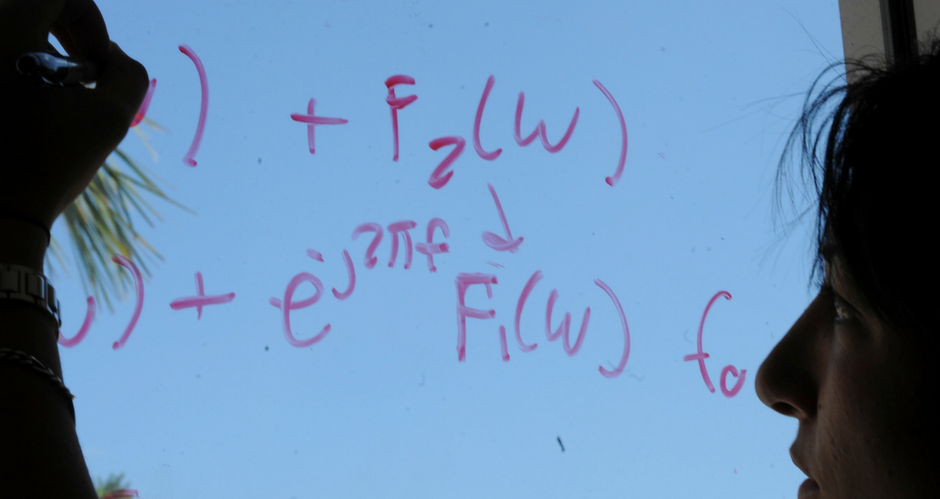## Mathematics and System Engineering Faculty Publications

### Existence to singular boundary value problems with sign changing nonlinearities using an approximation method approach

Article

#### Publication Title

Applications of Mathematics

#### Abstract

This paper studies the existence of solutions to the singular boundary value problem {−u′′=g(t,u)+(h,u),t∈(0,1),u(0)=0=u(1), {−u″=g(t,u)+(h,u),t∈(0,1),u(0)=0=u(1), , where g: (0, 1) × (0, ∞) → ℝ and h: (0, 1) × [0, ∞) → [0, ∞) are continuous. So our nonlinearity may be singular at t = 0, 1 and u = 0 and, moreover, may change sign. The approach is based on an approximation method together with the theory of upper and lower solutions.

117

135

#### DOI

10.1007/s10492-007-0006-5

4-2007

COinS